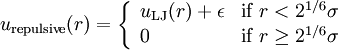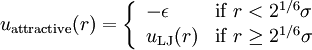Difference between revisions of "Weeks-Chandler-Andersen perturbation theory"$u_{\rm repulsive} (r) = \left\{ \begin{array}{ll} u_{\rm LJ}(r) + \epsilon & {\rm if} \; r < 2^{1/6}\sigma \\ 0 & {\rm if} \; r \ge 2^{1/6}\sigma \end{array} \right.$$u_{\rm attractive} (r) = \left\{ \begin{array}{ll} -\epsilon & {\rm if} \; r < 2^{1/6}\sigma \\ u_{\rm LJ}(r) & {\rm if} \; r \ge 2^{1/6}\sigma \end{array} \right.$